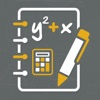Updated88888cc Calc
0 (0)
Pulled from iTunes
Wishlist
88888cc Calc
Marian Pearson
iPhone
Rating (Average):
0 (0)
Rating (Current):
0 (0)
Calc For Algebra is a tool to calculate math problem by equations. The main objective of these calculators is to assist students, professionals and researchers quickly perform such calculations easily.

This App Covers:
==============
Vector Subtraction Calculator
Angle Between Vectors Calculator
Complex Numbers Addition Calculator
Complex Numbers Subtraction Calculator
Complex Numbers Multiplication Calculator
Complex Numbers Division Calculator
Polynomial Subtraction Calculator
(a+b)^n Formula Expander